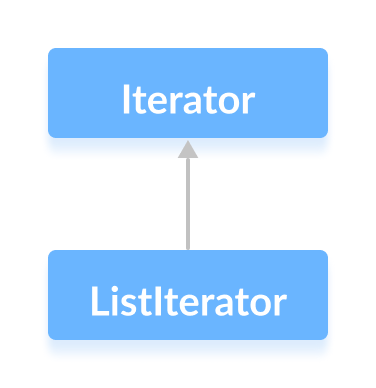# Java Iterator Interface

In this tutorial, we will learn about the Java Iterator interface with the help of an example.

The `Iterator` interface of the Java collections framework allows us to access elements of a collection. It has a subinterface `ListIterator`.All the Java collections include an `iterator()` method. This method returns an instance of iterator used to iterate over elements of collections.

## Methods of Iterator

The `Iterator` interface provides 4 methods that can be used to perform various operations on elements of collections.

• `hasNext()` - returns `true` if there exists an element in the collection
• `next()` - returns the next element of the collection
• `remove()` - removes the last element returned by the `next()`
• `forEachRemaining()` - performs the specified action for each remaining element of the collection

## Example: Implementation of Iterator

In the example below, we have implemented the `hasNext()`, `next(),` `remove()` and `forEachRemining()` methods of the `Iterator` interface in an array list.

``````import java.util.ArrayList;
import java.util.Iterator;

class Main {
public static void main(String[] args) {
// Creating an ArrayList
ArrayList<Integer> numbers = new ArrayList<>();
numbers.add(1);
numbers.add(3);
numbers.add(2);
System.out.println("ArrayList: " + numbers);

// Creating an instance of Iterator
Iterator<Integer> iterate = numbers.iterator();

// Using the next() method
int number = iterate.next();
System.out.println("Accessed Element: " + number);

// Using the remove() method
iterate.remove();
System.out.println("Removed Element: " + number);

System.out.print("Updated ArrayList: ");

// Using the hasNext() method
while(iterate.hasNext()) {
// Using the forEachRemaining() method
iterate.forEachRemaining((value) -> System.out.print(value + ", "));
}
}
}
``````

Output

```ArrayList: [1, 3, 2]
Acessed Element: 1
Removed Element: 1
Updated ArrayList: 3, 2,
```

In the above example, notice the statement:

``````iterate.forEachRemaining((value) -> System.put.print(value + ", "));
``````

Here, we have passed the lambda expression as an argument of the `forEachRemaining()` method.

Now the method will print all the remaining elements of the array list.

Did you find this article helpful?